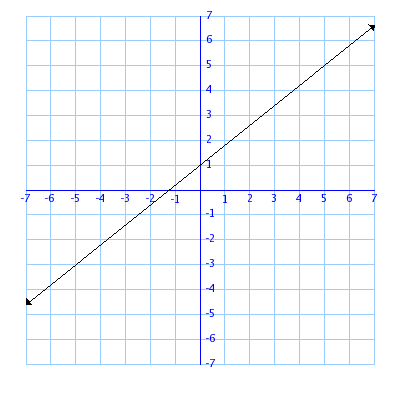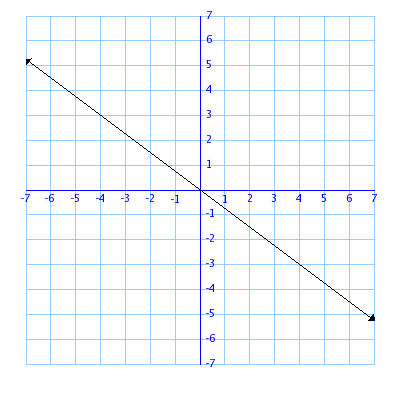# Math 10 – Week 12

In this week of math we learned an equation called rise/run or rise over run.

rise over run is an equation that is always used in graphing slopes. Now, slopes are the angle of what a line on a graph is.

in this picture, you can see that the Y = rise and X = runto find the slope on a graph first you have to find the rise. The rise is how many squares the distance from the bottom of the line to the top of the line.

next we find the run. The run is found by counting how long the line is. for example. In the photo above it shows 1 point at -1 and the other at -3. so the “Run” for this line is 2, because that is the distance horizontally of the line

next we put the rise over run into a fraction then just divide it. just like in the picture above. the rise is 4 so and the run is 2 so that would equal 2. The rise(Y) will always be on top and the run(X) will always be on the bottom.

these next two things are different types of slopes

Positive SlopeNegative Slope